# Tamilnadu Board Class 10 Maths Solutions Chapter 1 Relations and Functions Ex 1.3

## Tamilnadu State Board Class 10 Maths Solutions Chapter 1 Relations and Functions Ex 1.3

Question 1.
Let f = {(x,y)|x,y ∈ N and y = 2x} be a relation on N. Find the domain, co-domain and range. Is this relation a function?
Solution:
F = {(x, y)|x, y ∈ N and y = 2x}
x = {1,2,3,…}
y = {1 × 2, 2 × 2, 3 × 2, 4 × 2, 5 × 2 …}
R = {(1,2), (2,4), (3, 6), (4, 8), (5, 10),…}
Domain of R = {1, 2, 3, 4,…},
Co-domain = {1,2,3…..}
Range of R = {2, 4, 6, 8, 10,…}
Yes, this relation is a function.

Question 2.
Let X = {3, 4, 6, 8}. Determine whether the relation R = {(x,fx))|x ∈ X, f(x) = x2 + 1} is a function from X to N ?
Solution:
x = {3,4, 6, 8}
R = ((x,f(x))|x ∈ X,f(x) = X2 + 1}
f(x) = x2 + 1
f(3) = 32 + 1 = 10
f(4) = 42 + 1 = 17
f(6) = 62 + 1 = 37
f(8) = 82 + 1 = 65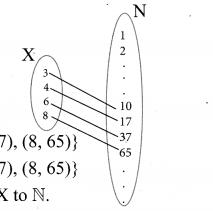R = {(3, 10), (4,17), (6,37), (8, 65)}
R = {(3, 10), (4,17), (6,37), (8, 65)}
Yes, R is a function from X to N.

Question 3.
Given the function f: x → x2 – 5x + 6, evaluate
(i) f(-1)
(ii) A(2a)
(iii) f(2)
(iv) f(x – 1)
Solution:
Give the function f: x → x2 – 5x + 6.
(i) f(-1) = (-1)2 – 5(1) + 6 = 1 + 5 + 6 = 12
(ii) f(2a) = (2a)2 – 5(2a) + 6 = 4a2 – 10a + 6
(iii) f(2) = 22 – 5(2) + 6 = 4 – 10 + 6 = 0
(iv) f(x- 1) = (x – 1)2 – 5(x – 1) + 6
= x2 – 2x + 1 – 5x + 5 + 6
= x2 – 7x + 12

Question 4.
A graph representing the function f(x) is given in figure it is clear that f (9) = 2.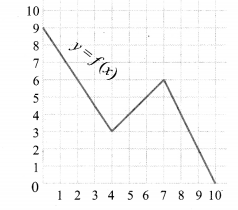(i) Find the following values of the function
(a) f(0)
(b) f(7)
(c) f(2)
(d) f(10)
(ii) For what value of x is f (x) = 1?
(iii) Describe the following
(i) Domain
(ii) Range.
(iv) What is the image of 6 under f?
Solution:
From the graph
(a) f(0) = 9
(b) f(7) = 6
(c) f(2) = 6
(d) f(10) = 0
(ii) Atx = 9.5, f(x) = 1
(iii) Domain = {0, 1, 2, 3, 4, 5, 6, 7, 8, 9, 10}
= {x |0 < x < 10, x ∈ R}
Range = {x|0 < x < 9, x ∈ R}
= {0, 1,2, 3,4, 5,6, 7, 8,9}
(iv) The image of 6 under f is 5.

Question 5.
Let f(x) = 2x + 5. If x ≠ 0 then findSolution:
Given f(x) = 2x + 5, x ≠ 0.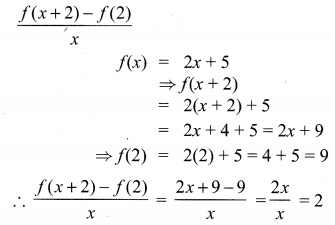Question 6.
A function fis defined by f(x) = 2x – 3
(i) find $$\frac{f(0)+f(1)}{2}$$
(ii) find x such that f(x) = 0.
(iii) find x such that f(x) = x.
(iv) find x such that f(x) = f(1 – x).
Solution:
Given f(x) = 2x – 3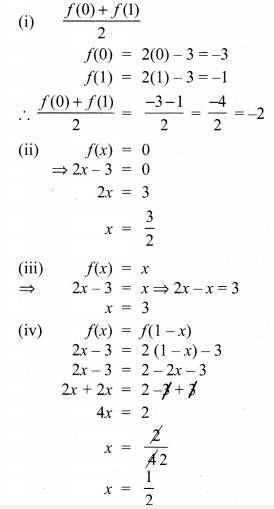Question 7.
An open box is to be made from a square piece of material, 24 cm on a side, by cutting equal squares from the corners and turning up the sides as shown in figure. Express the volume V of the box as a function of x.Solution:
Volume of the box = Volume of the cuboid
= l × b × h cu. units
Here l = 24 – 2x
b = 24 – 2x
h = x
∴ V = (24 – 2x) (24 – 2x) × x
= (576 – 48x – 48x + 4×2)x
V = 4x3 – 96x2 + 576x

Question 8.
A function f is defined bv f(x) = 3 – 2x . Find x such that f(x2) = (f(x))2.
Solution:
f(x) = 3 – 2x
f(x2) = 3 – 2x2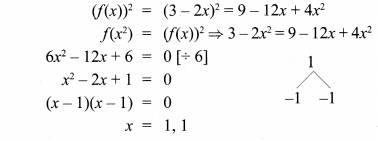Question 9.
A plane is flying at a speed of 500 km per hour. Express the distance d travelled by the plane as function of time t in hours.
Solution:Question 10.
The data in the adjacent table depicts the length of a woman’s forehand and her corresponding height. Based on this data, a student finds a relationship between the height (y) and the forehand length(x) as y = ax + b, where a, b are constants.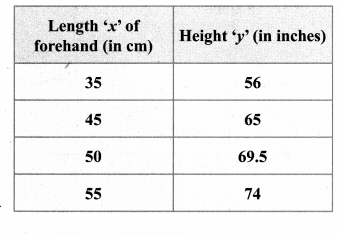(i) Check if this relation is a function.
(ii) Find a and b.
(iii) Find the height of a woman whose forehand length is 40 cm.
(iv) Find the length of forehand of a woman if her height is 53.3 inches.
Solution:
(i) Given y = ax + b …(1)
The ordered pairs are R = {(35, 56) (45, 65) (50, 69.5) (55, 74)}
∴ Hence this relation is a function.Substituting a = 0.9 in (2) we get
⇒ 65 = 45(.9) + b
⇒ 65 = 40.5 + b
⇒ b = 65 – 40.5
⇒ b = 24.5
∴ a = 0.9, b = 24.5
∴ y = 0.9x + 24.5
(iii) Given x = 40 , y = ?
∴ (4) → y = 0.9 (40) + 24.5
⇒ y = 36 + 24.5
⇒ y = 60.5 inches
(iv) Given y = 53.3 inches, x = ?
(4) → 53.3 = 0.9x + 24.5
⇒ 53.3 – 24.5 = 0.9x
⇒ 28.8 = 0.9x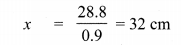∴ When y = 53.3 inches, x = 32 cm